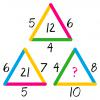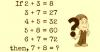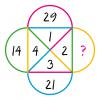BRAIN TEASERSBrain Teasers User Profile

# Srijegan Arulrajah

rank
333
points
8
See full ranking list
short ranking list
 331 KD P 8 332 Shehzad Raza 8 333 Srijegan Arulrajah 8 334 Anish Karki 8 335 Sherly Clement 8
 MATH PUZZLE: Can you replace... MATH PUZZLE: Can you replace the question mark with a number?Solve Math Puzzle If 2 + 3 = 8, 3 + 7 = 27, 4 + 5 = 32, 5 + 8 = 60, 6 + 7 = 72, then, 7 + 8 = ?Replace the question mark with a number MATH PUZZLE: Can you replace the question mark with a number?